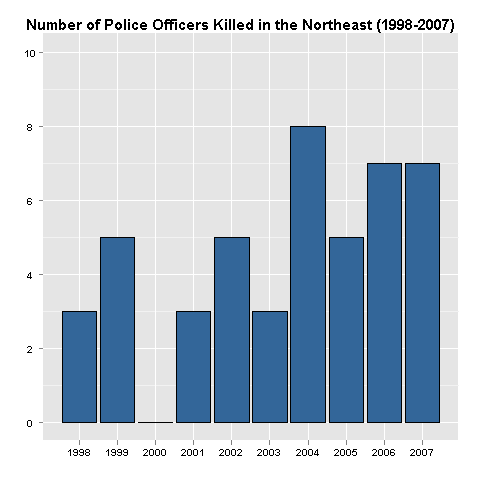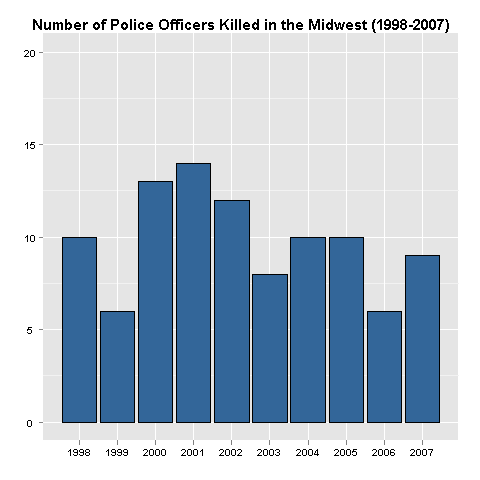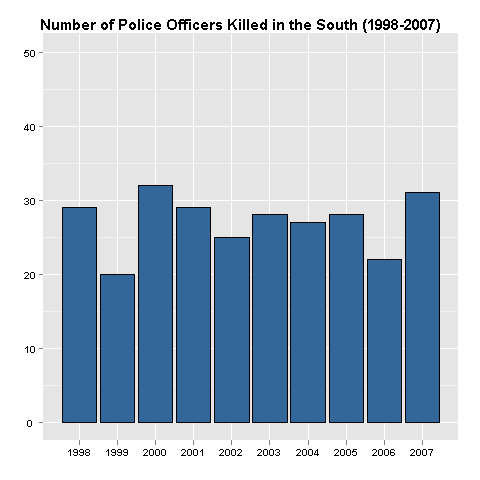Want to share your content on R-bloggers? click here if you have a blog, or here if you don't.

I would like to thank Tal Galili for establishing and maintaining the blog aggregator at R-bloggers. This site has been added to their directory and new posts which are tagged with R will now appear on their feed.

http://www.r-bloggers.com/

In part a, I presented a series of barplots which showed that the plurality of police officer killings from 1998 to 2007 occured in the southern region of the United States. Using the same data, I will examine whether there were any significant differences in the yearly number of officers killed in all four regions.```gplot(totnec, aes(Year, Value)) +
geom_bar(fill="#336699", colour="black") +
ylim(c(0,10)) + opts(title="Number of Police Officers Killed in the Northeast (1998-2007)") +
opts(axis.text.y=theme_text(family="sans", face="bold")) +
opts(axis.text.x=theme_text(family="sans", face="bold")) +
opts(plot.title = theme_text(size=15, face="bold")) +
xlab("") + ylab("")

ggplot(totmidc, aes(Year, Value)) + geom_bar() +
geom_bar(fill="#336699", colour="black") +
ylim(c(0,20)) + opts(title="Number of Police Officers Killed in the Midwest (1998-2007)") +
opts(axis.text.y=theme_text(family="sans", face="bold")) +
opts(axis.text.x=theme_text(family="sans", face="bold")) +
opts(plot.title = theme_text(size=15, face="bold")) +
xlab("") + ylab("")

ggplot(totsoc, aes(Year, Value)) + geom_bar() +
geom_bar(fill="#336699", colour="black") +
ylim(c(0,50)) + opts(title="Number of Police Officers Killed in the South (1998-2007)") +
opts(axis.text.y=theme_text(family="sans", face="bold")) +
opts(axis.text.x=theme_text(family="sans", face="bold")) +
opts(plot.title = theme_text(size=15, face="bold")) +
xlab("") + ylab("")

ggplot(totwec, aes(Year, Value)) + geom_bar() +
geom_bar(fill="#336699", colour="black") +
ylim(c(0,30)) + opts(title="Number of Police Officers Killed in the West (1998-2007)") +
opts(axis.text.y = theme_text(family="sans", face="bold")) +
opts(axis.text.x=theme_text(family="sans", face="bold")) +
opts(plot.title = theme_text(size=15, face="bold")) +
xlab("") + ylab("")```